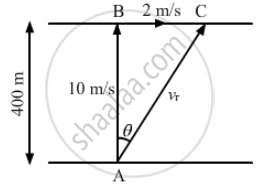Advertisement Remove all ads

# A river 400 m wide is flowing at a rate of 2.0 m/s. A boat is sailing at a velocity of 10 m/s with respect to the water, in a direction perpendicular to the river. How far from the - Physics

Sum

A river 400 m wide is flowing at a rate of 2.0 m/s. A boat is sailing at a velocity of 10 m/s with respect to the water, in a direction perpendicular to the river. How far from the point directly opposite to the starting point does the boat reach the opposite bank?

Advertisement Remove all ads

#### Solution

Given:
Distance between the opposite shore of the river or width of the river = 400 m
Rate of flow of the river = 2.0 m/s
Boat is sailing at the rate of 10 m/s.
The vertical component of velocity 10 m/s takes the boat to the opposite shore. The boat sails at the resultant velocity vr.
Time taken by the boat to reach the opposite shore:

$\text{ Time }= \frac{\text{ Distance } }{\text{ Time } } = \frac{400}{10} = 40 s$From the figure, we have:

$\tan \theta = \frac{2}{10} = \frac{1}{5}$
The boat will reach point C.
$\text{ In } ∆ ABC,$

$\tan \theta = \frac{BC}{AB} = \frac{BC}{400} = \frac{1}{5}$

$\Rightarrow BC = \frac{400}{5} = 80 \text{ m }$

Magnitude of velocity

$\left| v_r \right| = \sqrt{{10}^2 + 2^2} = 10 . 2 \text{ m/s }$

Let α be the angle made by the boat sailing with respect to the direction of flow.

$\tan\left( \alpha \right) = \frac{10}{2}$

$\Rightarrow \alpha = 78 . 7^\circ$

Distance the boat need to travel to reach the opposite shore = $\frac{400}{\sin\left( \alpha \right)} = 407 . 9 \text{ m }$

Using Pythagoras' theorem, we get:
Distance = $\sqrt{407 . 9^2 - {400}^2} = 79 . 9 \text{ m } \approx 80 \text{ m}$

Is there an error in this question or solution?
Advertisement Remove all ads

#### APPEARS IN

HC Verma Class 11, 12 Concepts of Physics 1
Chapter 3 Rest and Motion: Kinematics
Q 46.2 | Page 54
Advertisement Remove all ads
Advertisement Remove all ads
Share
Notifications

View all notifications

Forgot password?